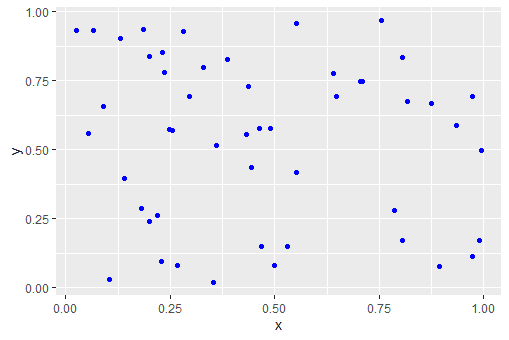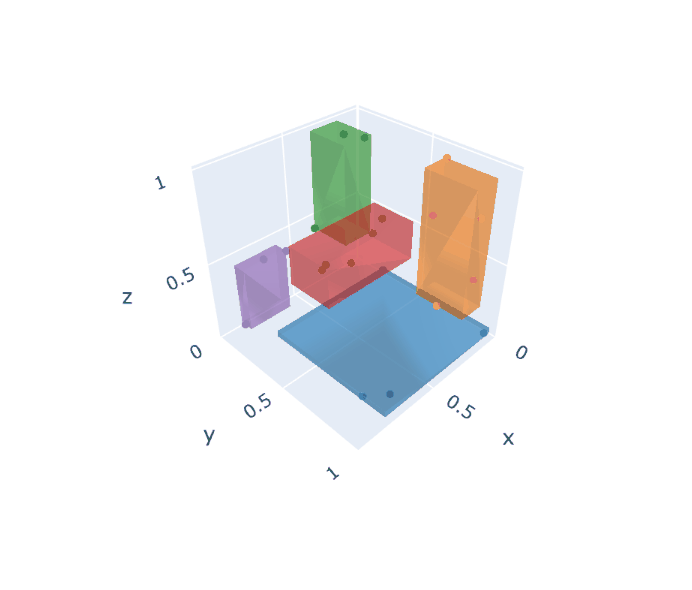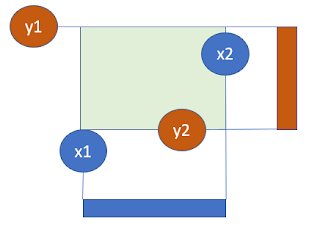## Rectangular Covering

This problem is from .

Problem statement: given $$N$$ data points, arrange $$k$$ boxes such that every point is inside a box and the size of the boxes is minimized. We assume the boxes have sides parallel to the axes. I.e. the box is not angled.

A 2d data set with $$N=50$$ points can look like:N=50 data points

The best configuration of $$K=5$$ boxes for this data set is:Optimal solution for K=5 rectangles

The problem is like the opposite of the "largest empty box" , where we try to find the largest box without any data points inside.

#### 2D Model

Let's define some symbols:

• We will denote the data points by $$i$$ and the rectangles by $$k$$.
• The coordinates of the data points are stored in $$p_{i,c} \in [L,U]$$ with $$c \in \{x,y\}$$.
• The location and size of the rectangles are $$r_{k,s}\in [L,U]$$ . Here $$s\in \{x,y,w,h\}$$.
• The assignment of (data) points to rectangles is modeled with a binary variable $$x_{i,k} \in \{0,1\}$$.

A high-level model for 2 dimensions can look like:

High-level 2D MIQP model
\begin{align}\min & \sum_k \color{darkred} r_{k,w} \cdot \color{darkred} r_{k,h} \\ & \sum_k \color{darkred}x_{i,k} = 1 && \forall i \\ & \color{darkred}x_{i,k}=1 \implies \begin{cases} \color{darkblue}p_{i,x} \ge \color{darkred}r_{k,x} \\ \color{darkblue}p_{i,y} \ge \color{darkred}r_{k,y} \\ \color{darkblue}p_{i,x} \le \color{darkred}r_{k,x}+\color{darkred}r_{k,w} \\ \color{darkblue}p_{i,y} \le \color{darkred}r_{k,y}+\color{darkred}r_{k,h} \end{cases} && \forall i,k\\ & \color{darkred} x_{i,k}\in \{0,1\} \\ & \color{darkred}r_{k,h} \in [\color{darkblue}L,\color{darkblue}U] \\ &\color{darkred}r_{k,x}+\color{darkred}r_{k,w} \le \color{darkblue}U \\ &\color{darkred}r_{k,y}+\color{darkred}r_{k,h} \le \color{darkblue}U \end{align}

The implication says "if $$x_{i,k}=1$$ then point $$i$$ should be in box $$k$$". This can be implemented using indicator constraints or with a big-M construct: \begin{align} & p_{i,x} \ge r_{k,x} -M(1-x_{i,k})\\ & p_{i,y} \ge r_{k,y}-M(1-x_{i,k}) \\ & p_{i,x} \le r_{k,x}+r_{k,w} +M(1-x_{i,k})\\ & p_{i,y} \le r_{k,y}+r_{k,h}+M(1-x_{i,k}) \end{align} A value for $$M$$ can be $$M=U-L$$. We can of course scale the data $$p_{i,c}$$ to $$p_{i,c} \in [0,1]$$  beforehand, in which case we can set $$M=1$$. This is what I did in my experiments.

I also add the constraint: $r_{k,x} \ge r_{k-1,x}$ to make the solution more predictable. The solution will be ordered by the $$x$$-coordinate of the box. It also reduces symmetry in the model.

The objective has non-convex quadratic terms. So we need a non-convex quadratic solver.

The detailed data and the solution look like:

----     56 PARAMETER p  data points

x           y

i1      0.80601     0.17283
i2      0.52952     0.14887
i3      0.64826     0.69240
i4      0.35222     0.02016
i5      0.43130     0.55356
i6      0.64087     0.77456
i7      0.23545     0.78074
i8      0.26800     0.08158
i9      0.97328     0.11440
i10     0.87431     0.66711
i11     0.75621     0.96771
i12     0.19863     0.24001
i13     0.21990     0.26101
i14     0.98863     0.17156
i15     0.06625     0.93008
i16     0.80603     0.83235
i17     0.10543     0.02902
i18     0.22927     0.09355
i19     0.12983     0.90322
i20     0.43682     0.72756
i21     0.24754     0.57512
i22     0.36012     0.51628
i23     0.71015     0.74601
i24     0.70367     0.74621
i25     0.18517     0.93583
i26     0.81681     0.67338
i27     0.46350     0.57754
i28     0.08932     0.65688
i29     0.97335     0.69059
i30     0.89449     0.07780
i31     0.14124     0.39550
i32     0.99382     0.49870
i33     0.05406     0.56047
i34     0.32927     0.79803
i35     0.22975     0.85134
i36     0.78532     0.27930
i37     0.18014     0.28874
i38     0.38628     0.82680
i39     0.48970     0.57654
i40     0.46709     0.14913
i41     0.19949     0.83893
i42     0.25438     0.57046
i43     0.55116     0.95672
i44     0.02634     0.93260
i45     0.49950     0.08040
i46     0.44529     0.43742
i47     0.55100     0.41666
i48     0.29626     0.69398
i49     0.93426     0.58556
i50     0.28083     0.92941

----     56 VARIABLE x.L  assignment variables

k1          k2          k3          k4          k5

i1                                                            1
i2                                    1
i3            1
i4                                    1
i5                                                1
i6            1
i7            1
i8                                    1
i9                                                            1
i10                                                           1
i11           1
i12                       1
i13                       1
i14                                                           1
i15           1
i16           1
i17                                   1
i18                                   1
i19           1
i20           1
i21                       1
i22                                               1
i23           1
i24           1
i25           1
i26                                                           1
i27                                               1
i28                       1
i29                                                           1
i30                                                           1
i31                       1
i32                                                           1
i33                       1
i34           1
i35           1
i36                                                           1
i37                       1
i38           1
i39                                               1
i40                                   1
i41           1
i42                       1
i43           1
i44           1
i45                                   1
i46                                               1
i47                                               1
i48           1
i49                                                           1
i50           1

----     56 VARIABLE r.L  rectangles

x           y           w           h

k1     0.02634     0.69240     0.77969     0.27530
k2     0.05406     0.24001     0.20032     0.41687
k3     0.10543     0.02016     0.42409     0.12897
k4     0.36012     0.41666     0.19087     0.16088
k5     0.78532     0.07780     0.20850     0.61279

----     56 VARIABLE z.L                   =      0.51132  objective


This model was solved to proven global optimality with Gurobi in about 670 seconds. Cplex also can solve models of this type.

#### To higher dimensions

The above model dealt with the 2D problem. It is not very difficult to extend this to more dimensions. Let's try to make this general, for any dimension.

First of all, I reorganized my sets a bit:

• We have $$d \in \{1,\dots,D\}$$ indicating the dimension we are looking at. Think about this as $$1=x,2=y,3=z,...$$.
• To characterize a box, for each dimension, we have a coordinate and a side-length. So, $$cs \in \{\mathrm{coord},\mathrm{side}\}$$.
With this, we can write:
• $$p_{i,d}$$ are the coordinates of the points,
• $$r_{k,d,cs}$$ indicate the location and size of the boxes.

The model can now look like:

High-level n-dimensional MINLP model
\begin{align}\min & \sum_k \prod_d \color{darkred} r_{k,d,side} \\ & \sum_k \color{darkred}x_{i,k} = 1 && \forall i \\ & \color{darkred}x_{i,k}=1 \implies \begin{cases} \color{darkblue}p_{i,d} \ge \color{darkred}r_{k,d,coord} \\ \color{darkblue}p_{i,d} \le \color{darkred}r_{k,d,coord}+\color{darkred}r_{k,d,side} \end{cases} && \forall i,k,d\\ & \color{darkred} x_{i,k}\in \{0,1\} \\ & \color{darkred}r_{k,d,cs} \in [\color{darkblue}L,\color{darkblue}U] \\ &\color{darkred}r_{k,d,coord}+\color{darkred}r_{k,d,side} \le \color{darkblue}U \end{align}

The objective is not quadratic. We can make it quadratic by chaining. We introduce a variable $$v$$ which is defined by:$v_{k,d} = \begin{cases} v_{k,d-1}\cdot r_{k,d,side} & \text{for d\ge 3}\\ r_{k,2,side} \cdot r_{k,1,side} & \text{for d=2}\end{cases}$ Now we can just minimize $$\sum_k v_{k,D}$$. For the special case of a 3d problem, we can simplify this to: \begin{align} \min & \sum_k \mathit{area}_k \cdot r_{k,3,side} \\ & \mathit{area}_k = r_{k,2,side}\cdot r_{k,1,side}\end{align}

A small data set ($$N=20$$ points, and $$K=5$$) leads to:

----     62 PARAMETER p  data points

dim1        dim2        dim3

i1      0.80601     0.17283     0.52952
i2      0.14887     0.64826     0.69240
i3      0.35222     0.02016     0.43130
i4      0.55356     0.64087     0.77456
i5      0.23545     0.78074     0.26800
i6      0.08158     0.97328     0.11440
i7      0.87431     0.66711     0.75621
i8      0.96771     0.19863     0.24001
i9      0.21990     0.26101     0.98863
i10     0.17156     0.06625     0.93008
i11     0.80603     0.83235     0.10543
i12     0.02902     0.22927     0.09355
i13     0.12983     0.90322     0.43682
i14     0.72756     0.24754     0.57512
i15     0.36012     0.51628     0.71015
i16     0.74601     0.70367     0.74621
i17     0.18517     0.93583     0.81681
i18     0.67338     0.46350     0.57754
i19     0.08932     0.65688     0.97335
i20     0.69059     0.89449     0.07780

----     62 VARIABLE x.L  assignment variables

k1          k2          k3          k4          k5

i1                                                            1
i2                        1
i3                                    1
i4                                                1
i5                        1
i6            1
i7                                                1
i8                                                            1
i9                                    1
i10                                   1
i11           1
i12           1
i13                       1
i14                                                           1
i15                                               1
i16                                               1
i17                       1
i18                                               1
i19                       1
i20           1

----     62 VARIABLE r.L  rectangles

dim1.coord   dim1.side  dim2.coord   dim2.side  dim3.coord   dim3.side

k1     0.02902     0.77701     0.22927     0.74401     0.07780     0.03660
k2     0.08932     0.14613     0.64826     0.28757     0.26800     0.70534
k3     0.17156     0.18066     0.02016     0.24086     0.43130     0.55733
k4     0.36012     0.51418     0.46350     0.24017     0.57754     0.19701
k5     0.72756     0.24015     0.17283     0.07471     0.24001     0.33511

----     62 VARIABLE z.L                   =      0.10539  objective


This model can be solved with Gurobi. Cplex does not allow non-convex quadratic constraints.3d solution

#### Linear formulation by set covering

This formulation was inspired by the comments by Rob Pratt below. The idea is to generate all possible rectangles in advance. We pick two arbitrary points to determine the horizontal part of the rectangle and two arbitrary points to determine the vertical part of the rectangle. This would mean ${50 \choose 2} \times {50 \choose 2}= 1,500,625$ rectangles. In addition, we need to keep track of which points are covered by each rectangle $$k$$. Let's introduce: $s_{i,k} = \begin{cases} 1 & \text{if point i is covered by rectangle k}\\ 0 & \text{otherwise}\end{cases}$ The resulting model is \begin{align} \min& \sum_k x_k \mathit{area}_k\\ & \sum_k x_k=5\\ & \sum_k s_{i,k} x_k \ge 1&& \forall i \\ & x_k \in \{0,1\} \end{align} This is now a very large, but very easy linear MIP model.

Notes:

• As we generate all rectangles in advance, we can also calculate the area (or volume) in advance. That explains why the model becomes linear.
• Note that the horizontal pair of points $$x_1,x_2$$ can be identical to the vertical pair $$y_1,y_2$$. They are drawn independently.
• We did not generate rectangles with just a single point. We can easily add them.
• When generating two "horizontal" points $$x_1, x_2$$ and two "vertical" points $$y_1,y_2$$, one or more of the points may be outside the resulting box. We can just drop that candidate rectangle. See the picture below. Note that elsewhere in the generation process we will form the rectangle: $$y_1,x_2$$ in the $$x$$ direction and $$x_1,y_1$$ in the $$y$$ direction.
• So: we generated more rectangles than needed if we did not drop cases like in the previous bullet. A little bit more logic is needed to prevent these unneeded rectangles. Note this will not change the solution. We just will generate a smaller model.
• I think if you do this right, then just 50,658 rectangles will be generated. This means we could discard 96.6% of the candidate rectangles!
• This can be extended to larger dimensions. I expect the total number of possible boxes can become very large, very quickly.This candidate rectangle is dropped from consideration.

#### References

1. How can I find k minimum bounding rectangles to enclose all the given points?, https://stackoverflow.com/questions/60401171/how-can-i-find-k-minimum-bounding-rectangles-to-enclose-all-the-given-points

1.Alternatively, you can model the problem linearly as a cardinality-constrained set covering problem, as follows. Let R be the set of all possible rectangles with a grid point on each boundary. Here, |R| = (50 choose 2)^2 = 1,500,625. For r in R, let binary variable U(r) indicate whether rectangle r is used. The objective is to minimize the total area sum {r in R} area(r) * U(r). The cardinality constraint is sum {r in R} U(r) = 5. The cover constraint for each point i is sum {r in R: i in R} U(r) >= 1. This formulation avoids the symmetry issue, and the resulting optimal solution matches yours.

1.Great idea. I think the number of rectangle is not 100% correct. We miss rectangles with a single point. Also some rectangles may not be of value, because one of the two "y" points (or both) may be outside the "x" window. And vice versa.

2.This comment has been removed by the author.

3.Yes, you can omit any rectangles that do not contain any points. In that case, I get 1,342,573 nonempty rectangles; I don't understand how to get 50,658. You can also allow side length 0, in which case I get 1,386,723 nonempty but possibly 0 area rectangles.

4.Yes, I confirm 50,658 rectangles now, including those with 0 area. I wrote "with a grid point on each boundary" but had not checked this condition.

5.This comment has been removed by the author.

6.For the 3D instance, I get 6039 useful boxes.

2.This comment has been removed by the author.